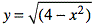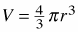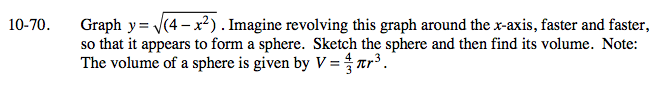### Home > A2C > Chapter 10 > Lesson 10.2.1 > Problem10-70

10-70.

Graph. Imagine revolving this graph around the x-axis, faster and faster, so that it appears to form a sphere. Sketch the sphere and then find its volume. Note: The volume of a sphere is given by. Homework Help ✎Square both sides of the first equation and convert it into graphing form. What is the radius of the sphere?

$\text{Volume }=\frac{32\pi}{3}$